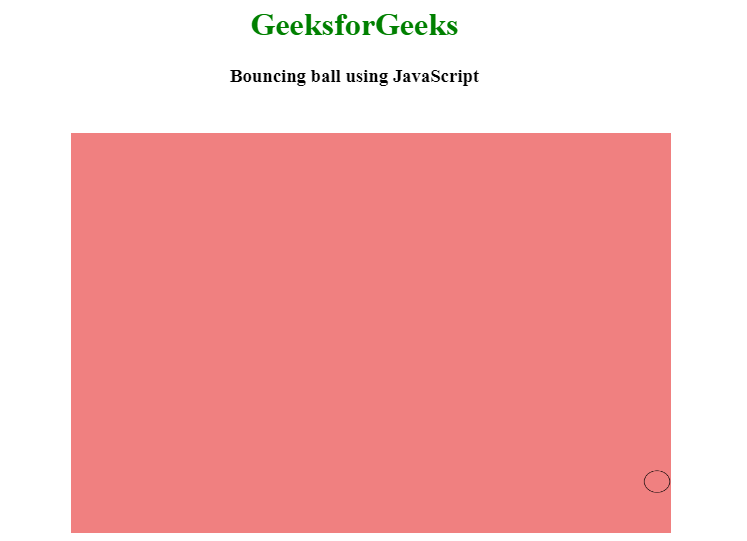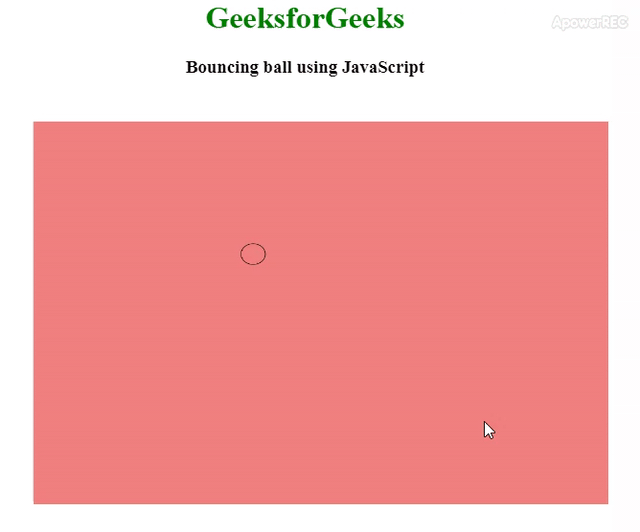Open in App
Not now

# How to Build a Bounce Ball with HTML and JavaScript ?

• Difficulty Level : Easy
• Last Updated : 25 Jan, 2023

A bouncing ball can be created by using HTML, CSS, and JavaScript and performing some bouncing operations on that ball. You can see a related article on how to make smooth bounce animation using CSS.

This article will be divided into two portions, 1st portion we will decide the area where the bouncing ball will perform bouncing, basically, we will create a canvas where bouncing will be performed. 2nd portion will design the bouncing ball and add some bouncing functionality to it.HTML & CSS: HTML and CSS code is used to create a canvas area where the ball will bounce. We will use a canvas tag and by using JavaScript we will struct the circle for the ball inside of that canvas. And the canvas area and background color of the canvas area is defined by the CSS.

JavaScript: It is the core part of this article where we will struct the ball and perform the bouncing task. We will assign 4 variables, 2 for the created circle(ball) coordinates and another two for the respective speed of the bouncing ball. The radius variable is used for the ball’s radius. We also need to clear the canvas area to do so we will use the clearReact() function. All the bouncing and coordination will be decided by the math.random() function.

Example:

## html

 ```<``html``>`` ` `<``head``>``    ``<``title``>``        ``Bouncing Ball!!``    ````    ``<``style``>``        ``h1 {``            ``color: green;``        ``}``         ` `        ``canvas {``            ``background-color: #F08080;``            ``width: 600px;``            ``height: 400px;``            ``position: absolute;``            ``top: 20%;``            ``left: 20%;``        ``}``    `````` ` `<``body``>``    ``<``center``>``        ``<``h1``>GeeksforGeeks``        ``<``h3``>Bouncing ball using JavaScript``        ``<``canvas``>``        ````    `````` ` ``

## Javascript

 ``My Personal Notes arrow_drop_up